# Data structure introduction

6 de Aug de 2018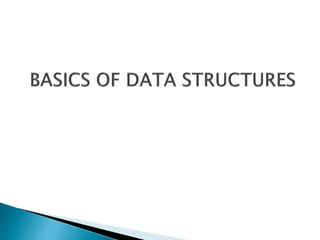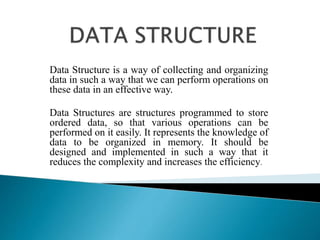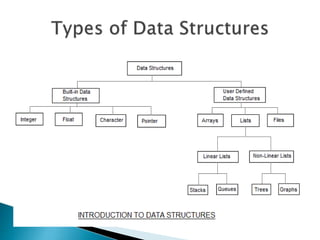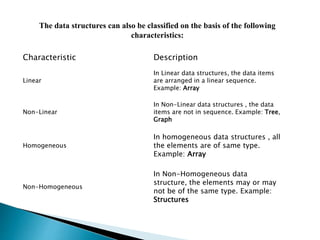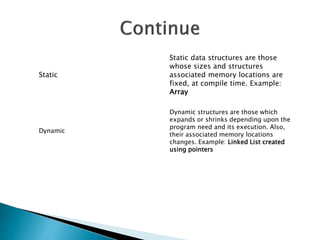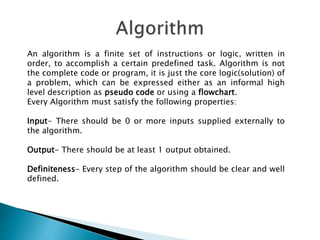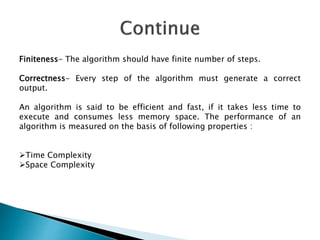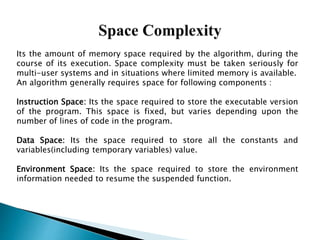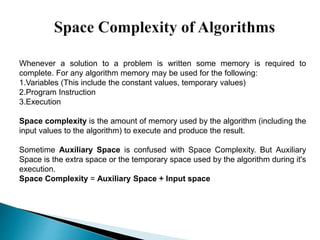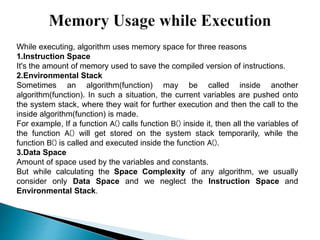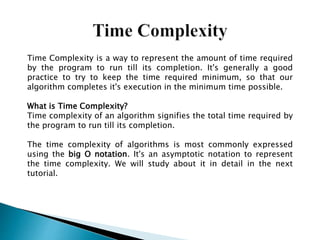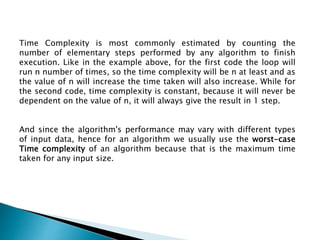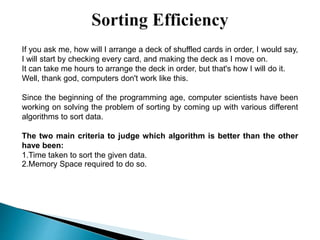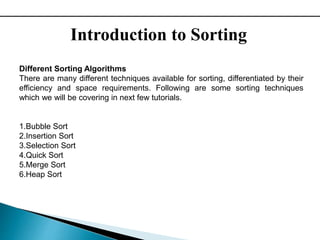1 de 14

### Data structure introduction

• 2. Data Structure is a way of collecting and organizing data in such a way that we can perform operations on these data in an effective way. Data Structures are structures programmed to store ordered data, so that various operations can be performed on it easily. It represents the knowledge of data to be organized in memory. It should be designed and implemented in such a way that it reduces the complexity and increases the efficiency.
• 4. Characteristic Description Linear In Linear data structures, the data items are arranged in a linear sequence. Example: Array Non-Linear In Non-Linear data structures , the data items are not in sequence. Example: Tree, Graph Homogeneous In homogeneous data structures , all the elements are of same type. Example: Array Non-Homogeneous In Non-Homogeneous data structure, the elements may or may not be of the same type. Example: Structures The data structures can also be classified on the basis of the following characteristics:
• 5. Static Static data structures are those whose sizes and structures associated memory locations are fixed, at compile time. Example: Array Dynamic Dynamic structures are those which expands or shrinks depending upon the program need and its execution. Also, their associated memory locations changes. Example: Linked List created using pointers
• 6. An algorithm is a finite set of instructions or logic, written in order, to accomplish a certain predefined task. Algorithm is not the complete code or program, it is just the core logic(solution) of a problem, which can be expressed either as an informal high level description as pseudo code or using a flowchart. Every Algorithm must satisfy the following properties: Input- There should be 0 or more inputs supplied externally to the algorithm. Output- There should be at least 1 output obtained. Definiteness- Every step of the algorithm should be clear and well defined.
• 7. Finiteness- The algorithm should have finite number of steps. Correctness- Every step of the algorithm must generate a correct output. An algorithm is said to be efficient and fast, if it takes less time to execute and consumes less memory space. The performance of an algorithm is measured on the basis of following properties : Time Complexity Space Complexity
• 8. Its the amount of memory space required by the algorithm, during the course of its execution. Space complexity must be taken seriously for multi-user systems and in situations where limited memory is available. An algorithm generally requires space for following components : Instruction Space: Its the space required to store the executable version of the program. This space is fixed, but varies depending upon the number of lines of code in the program. Data Space: Its the space required to store all the constants and variables(including temporary variables) value. Environment Space: Its the space required to store the environment information needed to resume the suspended function.
• 9. Whenever a solution to a problem is written some memory is required to complete. For any algorithm memory may be used for the following: 1.Variables (This include the constant values, temporary values) 2.Program Instruction 3.Execution Space complexity is the amount of memory used by the algorithm (including the input values to the algorithm) to execute and produce the result. Sometime Auxiliary Space is confused with Space Complexity. But Auxiliary Space is the extra space or the temporary space used by the algorithm during it's execution. Space Complexity = Auxiliary Space + Input space
• 10. While executing, algorithm uses memory space for three reasons: 1.Instruction Space It's the amount of memory used to save the compiled version of instructions. 2.Environmental Stack Sometimes an algorithm(function) may be called inside another algorithm(function). In such a situation, the current variables are pushed onto the system stack, where they wait for further execution and then the call to the inside algorithm(function) is made. For example, If a function A() calls function B() inside it, then all the variables of the function A() will get stored on the system stack temporarily, while the function B() is called and executed inside the function A(). 3.Data Space Amount of space used by the variables and constants. But while calculating the Space Complexity of any algorithm, we usually consider only Data Space and we neglect the Instruction Space and Environmental Stack.
• 11. Time Complexity is a way to represent the amount of time required by the program to run till its completion. It's generally a good practice to try to keep the time required minimum, so that our algorithm completes it's execution in the minimum time possible. What is Time Complexity? Time complexity of an algorithm signifies the total time required by the program to run till its completion. The time complexity of algorithms is most commonly expressed using the big O notation. It's an asymptotic notation to represent the time complexity. We will study about it in detail in the next tutorial.
• 12. Time Complexity is most commonly estimated by counting the number of elementary steps performed by any algorithm to finish execution. Like in the example above, for the first code the loop will run n number of times, so the time complexity will be n at least and as the value of n will increase the time taken will also increase. While for the second code, time complexity is constant, because it will never be dependent on the value of n, it will always give the result in 1 step. And since the algorithm's performance may vary with different types of input data, hence for an algorithm we usually use the worst-case Time complexity of an algorithm because that is the maximum time taken for any input size.
• 13. If you ask me, how will I arrange a deck of shuffled cards in order, I would say, I will start by checking every card, and making the deck as I move on. It can take me hours to arrange the deck in order, but that's how I will do it. Well, thank god, computers don't work like this. Since the beginning of the programming age, computer scientists have been working on solving the problem of sorting by coming up with various different algorithms to sort data. The two main criteria to judge which algorithm is better than the other have been: 1.Time taken to sort the given data. 2.Memory Space required to do so.
• 14. Introduction to Sorting Different Sorting Algorithms There are many different techniques available for sorting, differentiated by their efficiency and space requirements. Following are some sorting techniques which we will be covering in next few tutorials. 1.Bubble Sort 2.Insertion Sort 3.Selection Sort 4.Quick Sort 5.Merge Sort 6.Heap Sort